# Naming Of Bigger Compounds

## Naming Of Bigger Compounds

Having learnt the names of simple compounds we are now in happy situation to proceed to name the bigger or complex compounds by IUPAC system. There are however more than 70 rules in the system which guide us to name the organic compounds but here we are required to know only six basic rules.

1. Rule−1: Select the longest continuous carbon chain in a molecule whether it is in straight line (I) or not in straight line (II).
2.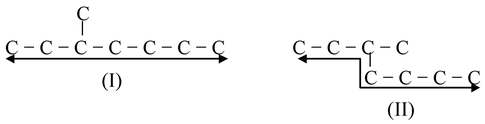3. Rule−2: If the compounds contains one or more longest continuous carbon chains then that chain is accepted which has the largest number of branches.
4. Let us understand it through an example,
5.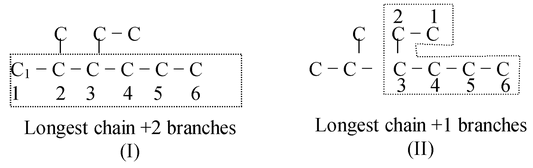6. The above two structural formulae are identical with, difference that if we view it in two ways (I) and (II). Both have the longest chains of 6 carbons atoms, which are numbered. In viewing as (I) we get two branches while viewed as (II) we get one branch.
7. So, (I) is the correct longest chain.
8. Rule−3: The rule states that after selecting the longest carbon chain, lowest position number is to be given to the position of substituent say, S. Let us have an example
9.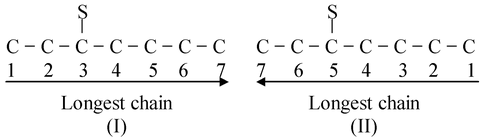10. In (I) 3 is the lower. Hence (I) is accepted and (II) is rejected. The positional number is also termed as LOCANT which means a number that locates the position of a substituent eg. Locant of S in (I)=3 and locant of S in (II)=5 due to incorrect numbering.
11. Rule−4: This rule is applicable to those compounds which have more than one substituent(S). In such cases counting is done from that terminal of carbon chain which gives lesser value for the sum of locant values of substituents. Such as
12.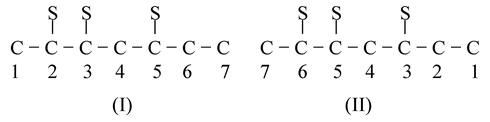13. 2 + 3 + 5 = 10 3 + 5 + 6 = 14
14. The compounds are identical and after counting we get 7C−atoms. But after counting, the sum of the locants of S should be lowest. Hence (I) is accepted for naming the compound.

<

### IUPAC names of compounds containing poly functional groups

 Functional groups Formula Name as substituent Name as parent Acid −COOH Carboxy Carboxylic or −oic   acid Sulphonic acid −SO3H Sulpho Sulphonic acid Ester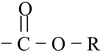Alkoxycarbonyl –oate Anhydride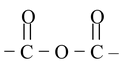– –oic anhydride Acid halide −COX Halo carbonyl –oyl halide Acid amide −CONH2 Carbamoyl or Amido Amide Cyanide −C ≡ N Cyano Nitrile Iso cyanide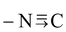Iso cyano Iso nitrile or carbyl   amine Aldehyde −CHO Oxo, aldo (for aldehyde)   Or formyl (for CHO) –al Ketone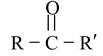Oxo or keto –one Alcohol −OH Hydroxy –ol Thiol –SH Mercapto Thiol Amines −NH2 Amino Amine Ethers −O− Alkoxy − Alkene = – ene Alkyne ≡ – yne Nitro. –NO2 Nitro – Nitroso. –N = O Nitroso – Halogen −X Halo − Alkyl –R Alkyl –